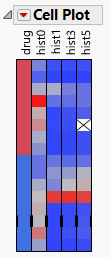Publication date: 08/13/2020

# The Cell Plot

To produce the plot shown in Figure 8.4, follow the instructions in Example of a Cell Plot.

Figure 8.4 The Cell Plot Report WindowNote: Any rows that are excluded in the data table are also hidden in the cell plot.

Cell plots are direct representations of a data table, drawn as a rectangular array of cells with each cell corresponding to a data table entry. Colors are assigned to each cell based on the range and type of values found in the column.

Nominal variables use a distinct color for each level. You can customize nominal and ordinal colors using the Value Colors property of data columns, available through the Column Info command.

Continuous variables are assigned a gradient of colors to show the smooth range of values in the variable.

Ordinal variables are scaled like continuous variables in order.

When some outliers are present, the scale uses all but the extreme categories for the 90% middle of the distribution, so that the outliers do not overly influence the scale.

The cell plot appears with a one-to-one correspondence of a colored cell representing each data table entry.

Related Information